# What is Martingale and Is It Reasonable to Use It?

### What Is Martingale?

If you write “martingale” in a search engine box, it will return a large number of pages with the description of this system. It is interesting that among others you will meet web-sites of online casinos, which assure that this system works, all you need is entering your credit card number to start scooping up money. What is strange – are the casinos ready to give their money such easily? If the Martingale really works so good, then why have not all the casinos turned bankrupt yet?

So, what is Martingale? Here is the definition from Wikipedia:

The Martingale is a betting system in gambling. The meaning is the following:

• A game starts with a certain minimal bet;
• After each each loss the bet should be increased so, that the win would recover all previous losses plus a small profit;
• In case of win a gambler returns to the minimal bet.

(Translated from Russian Wikipedia by MetaQuotes Software Corp.)

### Where Is Martingale Used?

The simplest gamble for analyzing the Martingale is chuck-farthing. The chances to win and to lose are equal – the gambler wins if a coin comes up heads and loses if the coin comes up tails. The Martingale system for this game works in such a way:

• Start the game with a small bet;
• After each loss double the bet;

The Martingale can also be used in playing the roulette, betting on red or black. The chances are less than 50/50, because there is also Zero, still very close to it.

As applied to trading, the following variant of the game can be used. Analogous to tossing a coin we open a position in any direction (short or long) with stop-loss and take-profit equally distant from the trade price. As we open the position in a random direction, the probability of profit and loss is analogous – 50/50. So in this article I will describe only the classical problem of tossing a coin with doubling the bet at a loss.

### Mathematical Part

Let us conduct a mathematical calculation of the dependence of the loss probability on the possible profit at the game with a coin using the Martingale system. Let us introduce the following symbols:

• Set – a set of tosses, ending by a winning one. I.e. all tosses except the last one are losing. At the first toss the bet is minimal, at each next toss in the set the bet is doubled;
• Q – initial deposit;
• – price of the starting bet;
• – maximal number of tosses (losing) in the set, leading to bankruptcy (suppose after k toss the deposit is equal to zero).

As we double the bet after each losing toss, we can derive the following equation: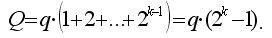Each set with the amount of tosses less than k-1 returns the profit q. As the probability of winning at a toss = ½, the average set length is 2*. Let us label by P(N) – the probability that we will not turn bankrupt within N tosses. As N tosses constitute approximately N/2 sets (the average set length is 2), and the probability to win in the set is (1/2)^k-1 , then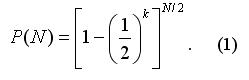We get the function of the win dependence on N. But the total number of tosses (N) is not informative enough, so let us try to bind N with an expected profit. Suppose, in the result we want to double our capital. As in set each we win q=Q/(2^k-1), the total profit is calculated according to the rule of the compound interest (more information about compound interest is here):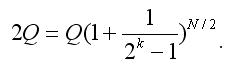After simple transformations we get the following formula for N: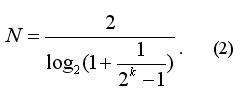After calculating the probability of the profit P(N) using the equities (1)-(2) we get the following results:
If we consider N a noninteger (do not round off the results of the equity (2) to a whole number), then P(N) does not depend on k and is equal to 1/2 (you can easily verify it, inserting (2) into (1) and using the simplest properties of logarithms). I.e. using the Martingale does not provide any advantages; we could as well bet all our capital Q and the winning probability would be the same (1/2).

### Conclusions of the Mathematical Part

Frankly speaking, at the beginning of preparing calculations for this article I expected that the Martingale would increase the probability of loss. It appeared to be wrong and the risk of loss is not increased. Still this article very vividly describes the meaninglessness of using the Martingale.

After getting the above formulas, the first thing I did was writing a small program, emulating the process of playing chuck-farthing and composing the statistics of the losing probability (P) dependence on the coefficient k. After the check I found that the program results (it can be called “an experiment”) coincide with mathematical calculations.
Of course, the ideal variant would be writing an Expert Advisor, trading by the same rules as in chuck-farthing and making sure that theoretical and experimental data are identical. But it is impossible because the starting bet is calculated using the formula: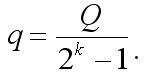And in the Forex we can “bet” only a sum multiple of 1/10 of a lot. That is why it is impossible to write an Expert Advisor, vividly proving the above formulas. Nevertheless, for completeness of analysis, we still can write an Expert Advisor, using the Martingale. But here the starting bet will be fixed – 0.1 of a lot. Analogous, the bet will be doubled at a loss and return to the starting one at profit. As described in the beginning of the article, a trade will be opened in the following way: a trade is opened in a random direction with the probability 50%, stoploss and takeprofit are fixed and equally distant.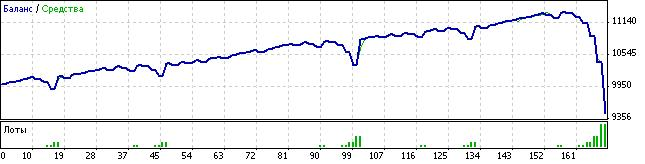The above screenshot displays the results of testing this Expert Advisor. You see, though the general direction of the curve is upwards, from time to time it suffers large dips. As a result of the last dip the Expert Advisor stops trading, because the balance is not enough for the next bet with a doubled lot. And at the moment of stop the balance is positive – here is the difference from the theoretical calculation in “the mathematical part”.

P.S. The files attached contain the screenshot of all necessary mathematical calculations and the Expert Advisor.